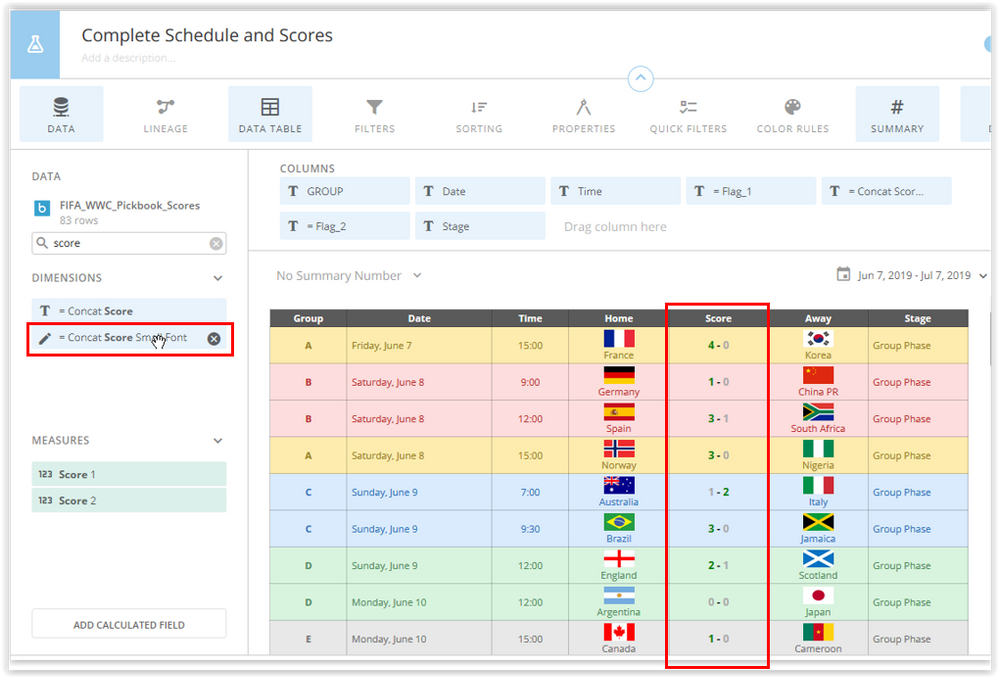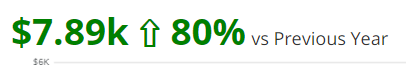# Summary Number - Conditional Format Colors with Directional Arrow

I've read a few posts here in the Dojo, and also read this Knowledge Base article: Sample Beast Mode Calculations: Card Element Transforms

but cannot get this to work.  It seems that the examples I am finding are far more complex than I need - maybe I'm missing something simple.

The logic I am trying to apply for a Summary Number is as follows:

CASE

WHEN `Gap` > 0 Then `Gap` [green text with green up arrow]

WHEN `Gap` < 0 Then `Gap` [red text with red down arrow]

ELSE `Gap` [black text with no arrow]

END

Here is a short video overview of what I'm asking for help with.

• Hi @swagner  , hope you are doing well

Take a look at this example, you will need to add some HTML code to your summary as well as aggregated (SUM, COUNT etc)

I have a field in a table that tells me the score of a soccer match

the code below bring the score and turns green for the winning team either on the left or the right and remains gray if there is a draw.

`Case when `Score 1` > `Score 2` then CONCAT('<div style="font-size:12px; color: Green;">', `Score 1`             ,'<span style="font-size:12px; color: Black;">', ' - '             ,'<span style="font-size:12px; color: DarkGray;">', `Score 2`, '</div>')     when  `Score 1` < `Score 2`  then CONCAT('<div style="font-size:12px; color: DarkGray;">', `Score 1`              ,'<span style="font-size:12px; color: Black;">', ' - '            ,'<span style="font-size:12px; color: Green;">', `Score 2`, '</div>')        when  `Score 1` = `Score 2`  then CONCAT('<div style="font-size:12px; color: DarkGray;">', `Score 1`              ,'<span style="font-size:12px; color: Black;">', ' - '             ,'<span style="font-size:12px; color: DarkGray;">', `Score 2`, '</div>') end  `

this is the final outcomeSame thing you would do for the summary number and to bring the image arrow uo or down , you will need the url of the image to render in the domo card. in the example above you can see some country flags and this is the code to combine the image with the country name

`CONCAT('<div ><img alt="TBA" height="20px" width="35"	src="',`Flag1`,'">','<br />' ,`Team 1`,'</div>')`

Domo Arigato!

**Say 'Thanks' by clicking the thumbs up in the post that helped you.
**Please mark the post that solves your problem as 'Accepted Solution'
• Hi swagner,

In order to do this you will need to use the concat function which will cause you to lose your summary number formatting.  Re-formatting the summary number is where the beast modes become very large.

The color and arrows can be accomplished by using this beast mode:

concat(

case

when `Gap`>0 then '<div style="color: green">'

when `Gap`<0 then  '<div style="color: red">'

else '<div style="color: black">'

end,

\$,

`Gap`,

case

when `Gap`>0 then ' &#8679; '

when `Gap`<0 then  ' &#8681; '

else ''

end,

'</div>')

Formatting the summary numbers with commas can be done by referencing this post.  I find it is easier to abbreviate the summary number rather than the full value but it depends on your use case.-----------------
Chris
• Posting for those that many follow this post later.  Below is the summary number beast mode I put together based on feedback here, adapted for \$ (the post @cwolman referenced, altered for my use case).  Includes the red/green color formatting, the directional arrow, and currency formatting.

`CASEWHEN (Sum(`Mayer`)-Sum(`Par`))<= 0 THENCase When Length(CONCAT(ROUND(Sum(`Mayer`)-Sum(`Par`),0),'')) = 9 THENCONCAT('<div style="color:red">','\$',SUBSTRING(CONCAT(Round((Sum(`Mayer`)-Sum(`Par`)),2),''),1,3),',',SUBSTRING(CONCAT(Round((Sum(`Mayer`)-Sum(`Par`)),0),''),3,3),',',SUBSTRING(CONCAT(Round((Sum(`Mayer`)-Sum(`Par`)),0),'' ),6,3), ' &#9660;','</div>')When Length(CONCAT(ROUND(Sum(`Mayer`)-Sum(`Par`),0),'')) = 8 THENCONCAT('<div style="color:red">','\$',SUBSTRING(CONCAT(Round((Sum(`Mayer`)-Sum(`Par`)),2),''),1,2),',',SUBSTRING(CONCAT(Round((Sum(`Mayer`)-Sum(`Par`)),0),''),3,3),',',SUBSTRING(CONCAT(Round((Sum(`Mayer`)-Sum(`Par`)),0),'' ),6,3), ' &#9660;','</div>')When Length(CONCAT(ROUND(Sum(`Mayer`)-Sum(`Par`),0),'')) = 7 THENCONCAT('<div style="color:red">','\$',SUBSTRING(CONCAT(Round((Sum(`Mayer`)-Sum(`Par`)),0),''),1,1),',',SUBSTRING(CONCAT(Round((Sum(`Mayer`)-Sum(`Par`)),0),''),2,3),',',SUBSTRING(CONCAT(Round((Sum(`Mayer`)-Sum(`Par`)),0),'' ),5,3), ' &#9660;', '</div>')When Length(CONCAT(ROUND(Sum(`Mayer`)-Sum(`Par`),0),'')) = 6 THENCONCAT('<div style="color:red">','\$',SUBSTRING(CONCAT(Round((Sum(`Mayer`)-Sum(`Par`)),0),''),1,3),',',SUBSTRING(CONCAT(Round((Sum(`Mayer`)-Sum(`Par`)),0),'' ),4,3), ' &#9660;','</div>')When Length(CONCAT(ROUND(Sum(`Mayer`)-Sum(`Par`),0),'')) = 5 THENCONCAT('<div style="color:red">','\$',SUBSTRING(CONCAT(Round((Sum(`Mayer`)-Sum(`Par`)),0),''),1,2),',',SUBSTRING(CONCAT(Round((Sum(`Mayer`)-Sum(`Par`)),0),'' ),3,3), ' &#9660;', '</div>')When Length(CONCAT(ROUND(Sum(`Mayer`)-Sum(`Par`),0),'')) = 4 THENCONCAT('<div style="color:red">','\$',SUBSTRING(CONCAT(Round((Sum(`Mayer`)-Sum(`Par`)),0),''),1,1),',',SUBSTRING(CONCAT(Round((Sum(`Mayer`)-Sum(`Par`)),0),'' ),2,3), ' &#9660;','</div>')When Length(CONCAT(ROUND(Sum(`Mayer`)-Sum(`Par`),0),'')) <= 3 THENCONCAT('<div style="color:red">','\$',Round(Sum(`Mayer`)-Sum(`Par`),0), ' &#9660;','</div>')ENDELSECase When Length(CONCAT(ROUND(Sum(`Mayer`)-Sum(`Par`),0),'')) = 9 THENCONCAT('<div style="color:green">','\$',SUBSTRING(CONCAT(Round((Sum(`Mayer`)-Sum(`Par`)),0),''),1,3),',',SUBSTRING(CONCAT(Round((Sum(`Mayer`)-Sum(`Par`)),0),''),3,3),',',SUBSTRING(CONCAT(Round((Sum(`Mayer`)-Sum(`Par`)),0),'' ),6,3), ' &#9650;','</div>')When Length(CONCAT(ROUND(Sum(`Mayer`)-Sum(`Par`),0),'')) = 8 THENCONCAT('<div style="color:green">','\$',SUBSTRING(CONCAT(Round((Sum(`Mayer`)-Sum(`Par`)),0),''),1,2),',',SUBSTRING(CONCAT(Round((Sum(`Mayer`)-Sum(`Par`)),0),''),3,3),',',SUBSTRING(CONCAT(Round((Sum(`Mayer`)-Sum(`Par`)),0),'' ),6,3), ' &#9650;','</div>')When Length(CONCAT(ROUND(Sum(`Mayer`)-Sum(`Par`),0),'')) = 7 THENCONCAT('<div style="color:green">','\$',SUBSTRING(CONCAT(Round((Sum(`Mayer`)-Sum(`Par`)),0),''),1,1),',',SUBSTRING(CONCAT(Round((Sum(`Mayer`)-Sum(`Par`)),0),''),2,3),',',SUBSTRING(CONCAT(Round((Sum(`Mayer`)-Sum(`Par`)),0),'' ),5,3), ' &#9650;','</div>')When Length(CONCAT(ROUND(Sum(`Mayer`)-Sum(`Par`),0),'')) = 6 THENCONCAT('<div style="color:green">','\$',SUBSTRING(CONCAT(Round((Sum(`Mayer`)-Sum(`Par`)),0),''),1,3),',',SUBSTRING(CONCAT(Round((Sum(`Mayer`)-Sum(`Par`)),0),'' ),4,3), ' &#9650;','</div>')When Length(CONCAT(ROUND(Sum(`Mayer`)-Sum(`Par`),0),'')) = 5 THENCONCAT('<div style="color:green">','\$',SUBSTRING(CONCAT(Round((Sum(`Mayer`)-Sum(`Par`)),0),''),1,2),',',SUBSTRING(CONCAT(Round((Sum(`Mayer`)-Sum(`Par`)),0),'' ),3,3), ' &#9650;','</div>')When Length(CONCAT(ROUND(Sum(`Mayer`)-Sum(`Par`),0),'')) = 4 THENCONCAT('<div style="color:green">','\$',SUBSTRING(CONCAT(Round((Sum(`Mayer`)-Sum(`Par`)),0),''),1,1),',',SUBSTRING(CONCAT(Round((Sum(`Mayer`)-Sum(`Par`)),0),'' ),2,3), ' &#9650;','</div>')When Length(CONCAT(ROUND(Sum(`Mayer`)-Sum(`Par`),0),'')) <= 3 THENCONCAT('<div style="color:green">','\$',Round(Sum(`Mayer`)-Sum(`Par`),0), ' &#9650;','</div>')ENDEND`

• I've got the following working, but the color formatting is not being applied.  Can you see what I'm missing:

`CONCAT(		CASE 			WHEN (SUM(`Mayer`)-SUM(`Par`))>0 THEN '<div style="color: green">'			WHEN (SUM(`Mayer`)-SUM(`Par`))<0 THEN '<div style="color: red">'			ELSE '<div style="color: black">'		END,		(SUM(`Mayer`)-SUM(`Par`))*100,  		'%',		CASE 			WHEN (SUM(`Mayer`)-SUM(`Par`))>0 THEN ' &#8679;'			WHEN (SUM(`Mayer`)-SUM(`Par`))>0 THEN ' &#8681;'			ELSE ''		END,'</div>')`
• @cwolman - nevermind... when I hit save & close it showed up with the format.  THANKS!

• @Godiepi thank you!  I will defintely use what you've posted here sir!

• @cwolman I'm having some trouble with this one. Using your suggested code, I'm getting the coloring to work but it's ending up creating a line break in my summary number.

Here is the format I want it to look like (albeit with color):

And here is what I'm getting:

And here is the code I'm using (abbreviated for space):

CONCAT(

'\$',

SUM('LY Rev'),

' Last Year, ',case when (ROUND(100*(SUM(`Rev`) - SUM(`LY Rev`) ) / (SUM(`LY Rev`)),2))>0 then '<div style="color: green">'

when (ROUND(100*(SUM(`Rev`) - SUM(`LY Rev`) ) / (SUM(`LY Rev`)),2))<0 then '<div style="color: red">'

else '<div style="color: black">'

end,

(ROUND(100*(SUM(`Rev`) - SUM(`LY Rev`) ) / (SUM(`LY Rev`)),2)),

'%', '</div>',

' Difference')

I don't understand why I'm getting the line breaks. Any help is appreciated.